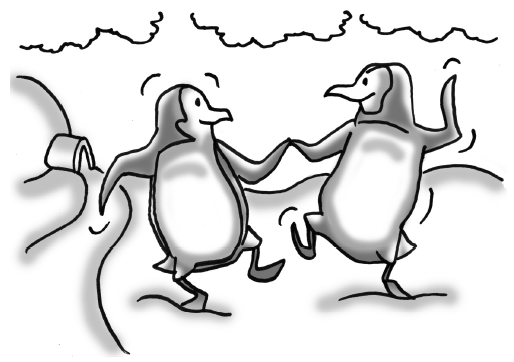### Home > GC > Chapter 3 > Lesson 3.1.4 > Problem3-41

3-41.Frank and Alice are penguins. At birth, Frank’s beak was $1.95$ inches long, while Alice’s was $1.50$ inches long. If Frank’s beak grows by $0.25$ inches per year and Alice’s grows by $0.40$ inches per year, how old will they be when their beaks are the same length?

Frank: $b=1.95+0.25y$ where $b=$beak length (in) $y$=time (years).
Use the same type of equation for Alice.

What must be true about the two equations if their beaks are the same length?

Solve the equation.